# How to calculate transcendental functions

There is sometimes need to calculate transcendental functions like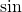,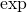,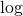or. We get them from the library and the library relies on implementations in the CPU for most of them. This is true, if we like to do them in „double“ format, which is the standard way of doing floating point arithmetic. But it can be interesting how these can be calculated to a given precision or to calculate functions that are not in the library and not easily composed from the library functions. There are many ways to do this and actually the naïve way of using the Taylor-series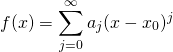is often not such a bad idea, if done correctly.
We know from math what to use for the coefficients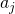and for which ranges of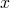this converges. For limited fixed precision it is possible to tune the coefficients a bit and get better results with a fixed number of summands. For arbitrary precision we need to be more flexible and cannot be prepared for this exact precision.

Now mathematically we can often have a converging series, for example if we haveThis converges for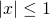, but the convergence is not necessarily computer friendly. It can be proved easily, that this series converges for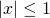, but forit converges slowly. To give an idea, if we are calculating with 100 digits after the decimal point then we would still have single terms in the area of our desired precision for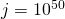and since they get smaller only slowly, we would have to go much further. This is impossible to use.

As a rule of thumb the coefficients are not our friends. They may or may not converge towards zero, but we really have to rely on the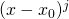-part to get diminishing summands. A good idea is to consider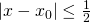if the coefficients are bounded, which they usually are in real life examples. That means that there is a boundary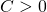such that for eachwe have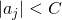. So we absolutely need to use some mathematical knowledge about the function in order to get reasonable convergence.

In case of periodic functions like the trigonometric functions, we can normalize x to values within one „period“, but that will reduceoronly to a range of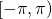. Using some common trigonometric formulas, we can actually reduce this to the range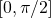, which is still not good enough. In this case we have to use formulas likeand similar formulas for other trigonometric functions. These allow us to move to smaller values of. For the exponential function, we have even easier ways. Let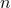be a natural number such that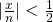. Then we let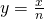and we can calculate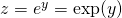. Now we have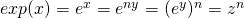and we just need to take the-th power of the intermediate result. This can be calculated using algorithms like square and multiply or even some improvements over that.

In the end we will end up writing a lot of code for different cases which are optimized in different ways for some function. For example the power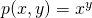is a function in two parameters, that has quite a wild behavior and for writing an implementation that provides reasonable performance and precision we need to handle a lot of cases. Just look at the power function of the standard Java library, which is written in native C-code. Its beauty is not the conciseness, but having some understanding about what it takes to do this well you might eventually appreciate the given implementation, even if you not only use it, but also read it.

Now dealing with the precision is a delicate question, which again requires mathematics. As a general rule we usually need to use more precision for intermediate results. A good tool is to take the derivative or the partial derivatives in case of functions with multiple parameters to see how much changes in that parameter influence changes of the value. The Taylor theorem gives some definite, but possibly hard to apply answers. And it can also be useful to look at lower and upper bounds for the operations performed.

When writing such functions, unit tests are a big deal. Often they are not so hard to write, if we have inverse functions to rely on or if we can increase the precision and see that the lower precision is at least as precise as it claims to be. In some cases existing implementations for double can be used to check if the calculation is correct for smaller precisions.

Most of all it is important to think and use some mathematics or get help for this from somebody with appropriate knowledge.

Just to give you a hint: There are tons of transcendental functions that do not exist in standard libraries and that may be interesting to use. For some of them there are libraries. For some we still need to find libraries or write them.